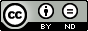#Analyzing the identifiability of sparse linear networks by Léon Zheng (ENS de Lyon) [June 28, 2021]

## Summary

GdR ISIS Théorie du deep learning - June 28, 2021

# By Léon Zheng (ENS de Lyon)

Sparsity in deep neural networks is desired for reducing time and space complexity of the model. It can also be considered in the hope of making the learned model interpretable. In particular, such interpretability would require some kind of stability or identifiability of the parameters. Although identifiability is well-understood in linear inverse problems regularized by sparsity, things are different in the case of networks with multiple sparse layers. We study here identifiability of sparse linear neural networks with two layers, and show some consequences of the analysis to the multilayer case.
We give conditions under which the problem of factorizing a matrix into two sparse factors admits a unique solution, up to unavoidable equivalences. Our framework considers an arbitrary family of sparsity patterns, allowing us to capture more structured notions of sparsity than simply the count of nonzero
entries. These conditions are shown to be related to the uniqueness of exact matrix decomposition into rank-one matrices, with sparsity constraints. Simple sufficient conditions for identifiability can be derived from this framework, which are verified for instance by the DCT and DST matrices of size N = 2L, when enforcing N -sparsity by column on the left factor, and 2-sparsity by row on 2 the right factor. Our analysis can then be extended to the multilayer case, as we give a formal proof that the DFT matrix of size 2L admits a unique sparse factorization into L factors, when enforcing the butterfly supports as the sparsity constraints on the L factors.

This is a cowork by Tung Le Quoc, Rémi Gribonval, Elisa Riccietti.

## Infos

• ### Updated on:

June 29, 2021, 8:19 p.m.

00:17:45

9

French

Other
• ### Discipline(s):